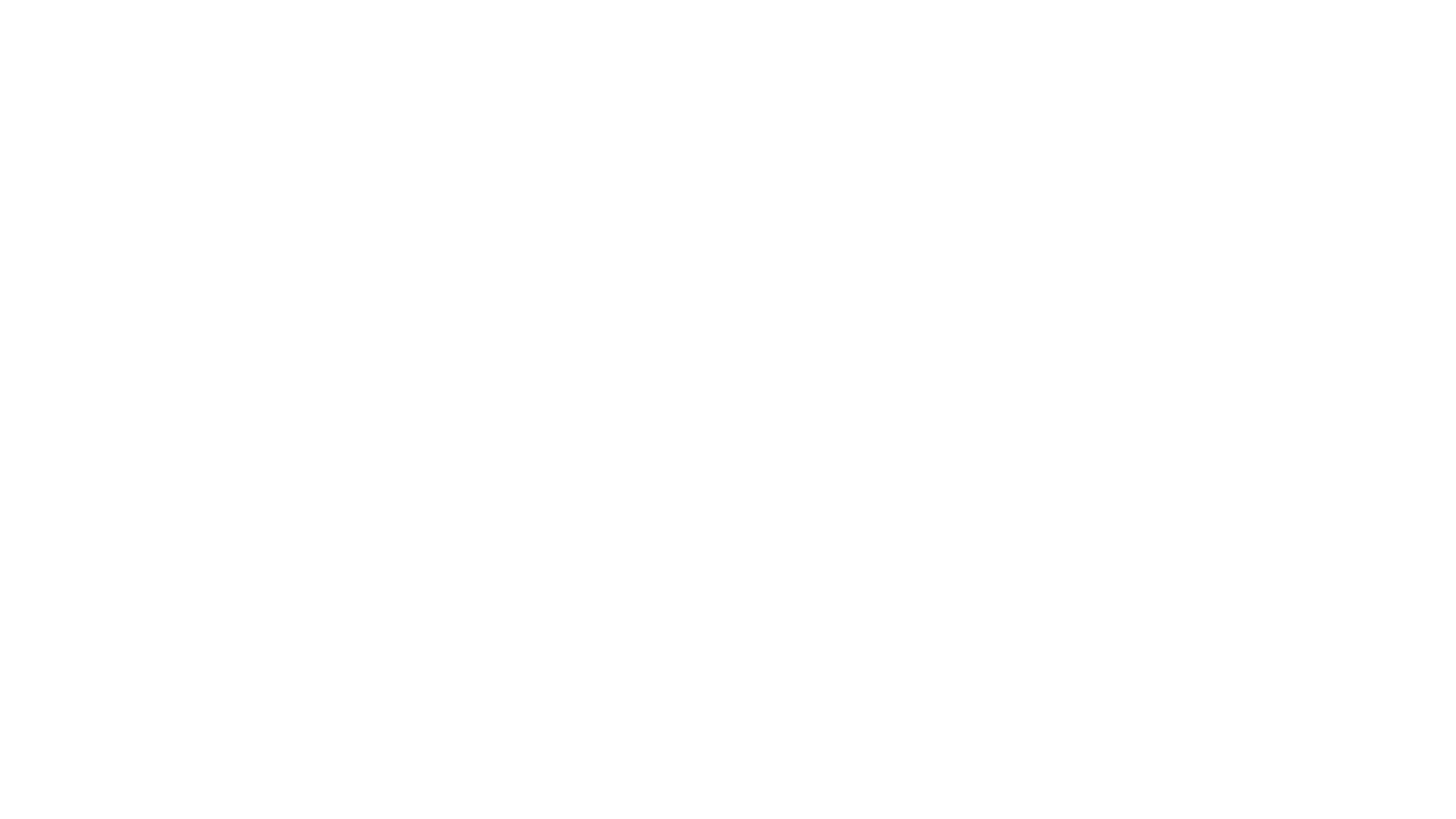1. 字符串（String）、数字(Number)、布尔(Boolean)、对空（Null）、未定义（Undefined）、Symbol。
2. 对象(Object)、数组(Array)、函数(Function)。

1. 参数通过值传递
• 这种情况下，如果函数改变了参数的值，它不会改变参数的原始值。
2. 参数通过引用传递
• 这种情况下，如果函数改变了参数的值，原始值也会改变。

``````//值传递
var num = 1;
function xx(arg){
arg=2;
}
xx(num);
console.log(num);//1``````
``````//对象
var a = {b:1};
function xx(obj){
obj.b=2;
}
xx(a);
console.log(a);//{b: 2}
``````
``````
//数组
var arr = [1,2];
function xx(obj){
obj=2;
}
xx(arr);
console.log(arr);// [2, 2]``````

``````
//这里注意观察与上面对象代码块的不同
var a = {b:1};
function xx(obj){
obj = {x:2};
}
xx(a);
console.log(a);//{b: 1}``````